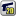﻿ Unreal Software - Thread: Knockback function

# ForumCS2DScriptsKnockback function

#Knockback function

15 replies
Goto Page1Mami Tomoe
User
OfflineHold on! I did search the forum, all I found were complete scripts for different purposes that don't work as I intend.

They either used arrays, that I don't want or they had hard to configure (or bad) weapon system.
They also didn't let me add more than just weapons very easily.

Hello!
I'm in the lookout for a function that receives the following parameters:
(p, angle, distance)

It will knock back the player
p
to the distance
distance
using the angle
angle
.

That's all I need, I can (probably) make the rest of the calculations alone.

Note: It shouldn't let a player enter somewhere he can't get out of.
Note: It should be efficient (no arrays please).
Note: Distance should be in pixels, of course.

Thank you!uaiek
User
OfflineFor simple implementation, assume the player is at pixel coordinates (px,py). Use setpos to set the player's position to (px+distance*cos(angle), py+distance*sin(angle)). However, if you don't want the player to be pushed, say, inside a wall, then you should do ray tracing method, for example (pseudocode):

Code:
1
2
3
4
5
6
7
8
9
10
11
dir := normalize(cos(angle), sin(angle))
final_target := (px, py)

for t from 0 to distance step by s:
tentative_target := (px,py) + dir * t
if tile at tentative_target is walkable:
final_target := tentative_target
else:
break

setpos(p, final_target)

Set the step value s to not too small or not too big (like distance/10) will achieve correct result with good performance.
edited 2×, last 17.09.19 01:06:24 pm
It seems to be good.
Mami Tomoe
User
OfflineThat is Lua right...?Devil-Thanh
GAME BANNED
Offlinethat's mostly like pascaluaiek
User
OfflineMami Tomoe has written:
That is Lua right...?No, it's not. That is a piece of pseudo code to demonstrate the algorithm. You said you can do the rest of the calculations alone so I thought you can implement it in Lua by yourself.
It seems to be good.
Mami Tomoe
User
OfflineI program in C# primarily, I don't know what a "pseudo" is.

I meant if you give me the function in Lua, I can make use of it from there.

I don't need weapon config or the other stuff that people usually post.
Mami Tomoe
User
OfflineCode:
1
2
3
4
5
6
7
8
local function knockback(p, angle, distance)
local x, y = get_player(p, 'x'), get_player(p, 'y')
local new_x, new_y = x + distance * math_cos(angle), y + distance * math_sin(angle)

if tile(math_ceil(new_x / 32), math_ceil(new_y / 32), 'walkable') then
exec('setpos ' .. p .. ' ' .. new_x .. ' ' .. new_y)
end
end

I've created this, is there any better way of doing it?
The
if
line I mean.

@Gaios: Pay attention to what I need above, those threads/files provide inefficient and overkill scripts, some even being outdated.
uaiek
User
OfflineThis is near correct, but If the tile is not walkable, the player won't be knocked back at all. I think you'd like to have the player pushed to the edge of the unwalkable tile.
It seems to be good.
Mami Tomoe
User
OfflineDo you know a good way of doing it? I tried using a while loop but it was quite messy.

First the game froze in an infinite loop, then it made it possible to go over walls...

It was that point I decided to give up until I have to do it alone or someone can help.Gaios
Security Supporter
Offline@Mami Tomoe: You need to do some precision, eg. each 5px.

This is some stuff that I use:
Code:
1
2
3
4
5
6
7
8
9
function pos_trigger(x, y, rot, power)
rot = (rot < -90) and rot + 360 or rot

local angle = math.rad(math.abs(rot + 90)) - math.pi
local new_x = math.floor((x + math.cos(angle) * power))
local new_y = math.floor((y + math.sin(angle) * power))

return new_x, new_y
end

This may be optimized to local vars eg.
local floor = math.floor
but in global scope.
Mami Tomoe
User
Offline@Gaios: I don't exactly understand what your function is used for...

EDIT:
Okay, now I get it.

Nice code! With a bit of tinkering I got it to a 95% perfection state!

Code:
1
2
3
4
5
6
7
8
9
10
11
12
13
local function knockback(p, rot, power)
local x, y = get_player(p, 'x'), get_player(p, 'y')

rot = (rot < -90) and rot + 360 or rot

local angle = math_rad(math_abs(rot + 90)) - math_pi
local new_x = math_floor((x + math_cos(angle) * power))
local new_y = math_floor((y + math_sin(angle) * power))

if tile(math_floor(new_x / 32), math_floor(new_y / 32), 'walkable') then
exec('setpos ' .. p .. ' ' .. new_x .. ' ' .. new_y)
end
end

One problem though...

This area is not walk-able, as you can see the bot can't get out:But in the console I get this:edited 1×, last 18.09.19 12:40:34 am
Gaios
Security Supporter
Offline@Mami Tomoe: You may do at least triple line checking. Player colision size is 20x20 px.
Mami Tomoe
User
Offline@Gaios: thanks it works now.

EDIT:

I've come across a problem where a power above 32~ tiles will make the player skip the wall, which can result in no-clipping outside of the map.

Is there a good fix for that?
edited 1×, last 21.09.19 07:44:12 pm
Gaios
Security Supporter
Offline@Mami Tomoe: If we assume that
power
won't be greater than 60 then just divide the power by 2 and do double check for normal power and divided one.
Mami Tomoe
User
OfflineAfter a couple of overflows it seems to work, thanks.1﻿